• 2019-01-16 10:57:53

Synopsys的程序包STD_LOGIC_ARITH、STD_LOGIC_SIGNED和STD_LOGIC_UNSIGNED中已经为许多类型的运算重载了算数运算符合关系运算符，因此只要引用这些程序包，SIGNED、UNSIGNED、STD_LOGIC、STD_LOGIC_VECTOR之间也可以混合运算。
但执行加法时，同时调用STD_LOGIC_SIGNED和STD_LOGIC_UNSIGNED程序会报错，把STD_LOGIC_SIGNED屏蔽之后又可以了。加法 关系运算符重载
更多相关内容
• Numpy中的std计算与课本中的计算方式相同，都是除的是样本数量n。 Torch中的std计算默认使用的是unbiased=True即贝塞尔校正，除的是样本数量n-1。

# 输入：

[1.0000, -1.0000, 3.0000]


# 课本中的标准差计算公式：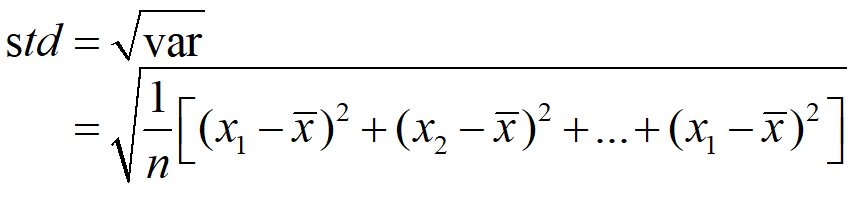按照上述公式计算：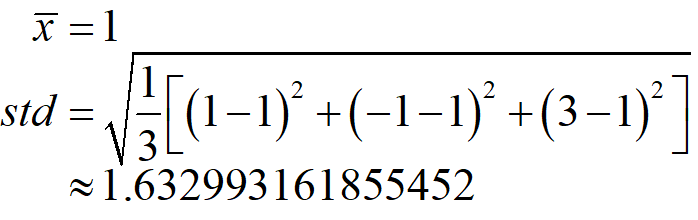# Numpy中的std计算：

import numpy as np

tm = np.array([1.0000, -1.0000, 3.0000])
ddd = np.std(tm)
print(ddd)

1.632993161855452


可以看出Numpy中的计算结果与课本中的公式计算出来的结果是一致的。

# Torch中的std计算：

tm = torch.tensor([1.0000, -1.0000, 3.0000])
ddd = torch.std(tm)
print(ddd)

tensor(2.)


计算出来的结果是2，与Numpy中的计算结果是不相同的。
查看torch.std的参数：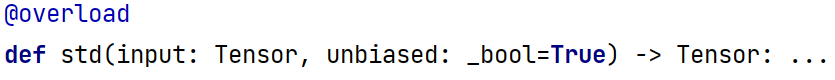torch.std默认设置了unbiased=True。此时计算标准差的公式则使用贝塞尔校正 的方法：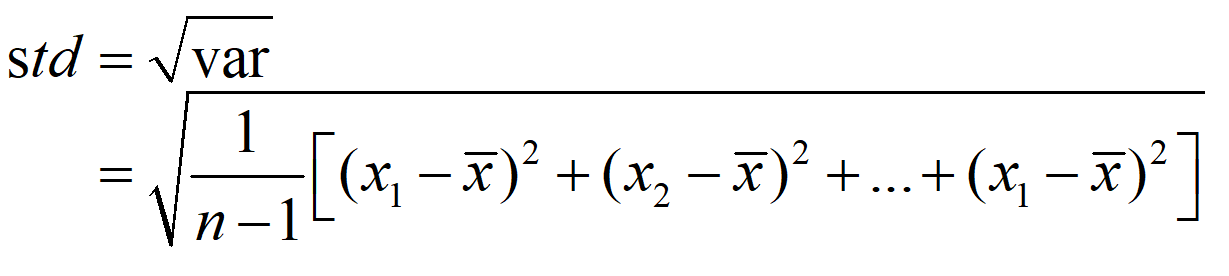可以看出贝塞尔校正的标准差最后除以n - 1。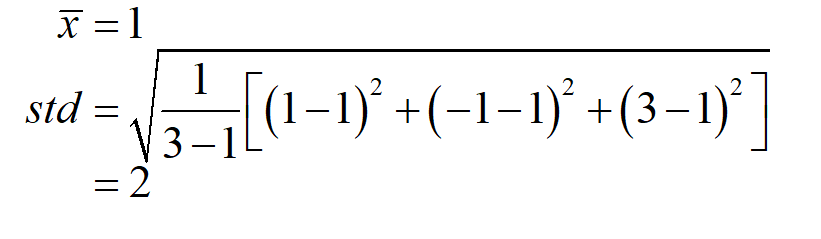可以看出确实计算出来的结果是2.
至于为何使用n-1，这里不做过多介绍，建议参考：贝塞尔校正
注：设置torch.std中的unbiased=False，则与Numpy中的std的结果相同的。

# 总结：

Numpy中的std计算与课本中的计算方式相同，都是除的是样本数量n。
Torch中的std计算默认使用的是unbiased=True即贝塞尔校正，除的是样本数量n-1。

展开全文pytorch Numpy Python 数学
• np.std()函数被用来：计算沿指定轴的标准差。返回数组元素的标准差 例子： import numpy as np a = np.array([[1, 2], [3, 4]]) print(np.std(a)) # 计算全局标准差 1.118033988749895 print(np.std(a, axis=0)) ...

np.std()函数被用来：计算沿指定轴的标准差。返回数组元素的标准差

例子：

import numpy as np
a = np.array([[1, 2], [3, 4]])
print(np.std(a))            # 计算全局标准差 1.118033988749895
print(np.std(a, axis=0))    # axis=0计算每一列的标准差 [1. 1.]
print(np.std(a, axis=1))    # axis=1计算每一行的标准差 [0.5 0.5]


展开全文python 矩阵
• 遇到这个问题可能就是版本较低出现问题，我的springboot parent版本为2.3.2.RELEASE <parent> <groupId>org.springframework.boot</groupId> <artifactId>spring-boot-starter-parent&...

遇到这个问题可能就是版本较低出现问题，我的springboot parent版本为2.3.2.RELEASE

<parent>
<groupId>org.springframework.boot</groupId>
<artifactId>spring-boot-starter-parent</artifactId>
<version>2.3.2.RELEASE</version>
<relativePath/> <!-- lookup parent from repository -->
</parent>


springboot的web模块和2.9.5版本的jackson-databind一起使用会报错

<dependency>
<groupId>com.fasterxml.jackson.core</groupId>
<artifactId>jackson-databind</artifactId>
<version>2.9.5</version>
</dependency>


将版本改为2.10.1即可

<dependency>
<groupId>com.fasterxml.jackson.core</groupId>
<artifactId>jackson-databind</artifactId>
<version>2.10.1</version>
</dependency>

展开全文java
• ## Numpy入门(十一)：np.std()用法

万次阅读 多人点赞 2020-10-08 14:48:35
numpy.std(a, axis=None, dtype=None, out=None, ddof=0) a： array_like，需计算标准差的数组 axis： int, 可选，计算标准差的轴。默认情况是计算扁平数组的标准偏差。 dtype： dtype, 可选，用于计算标准差的类型...python numpy
• pandas中std和numpy的std区别 pandas中Series.std的官方文档 numpy中numpy.std的官方文档 原理     计算标准差时，需要注意numpy中的std和pandas的std在计算标准差时，默认的计算结果会存在不一致的问题。  ...python numpy
• 刚刚写了一个返回Map的方法，...at com.fasterxml.jackson.databind.ser.std.MapSerializer.serialize(MapSerializer.java:536) at com.fasterxml.jackson.databind.ser.std.MapSerializer.serialize(MapSerializer.jav
• ## 标准差np.std()

千次阅读 2019-02-23 13:38:43
定义：标准差（Standard Deviation），能反映一个数据集的离散程度。平均数相同的两组数据，标准差未必相同。由于方差是数据的平方，与检测值本身相差太大，人们难以直观的衡量，所以常用方差开根号换算回来这就是...标准差
• numpy求均值、方差、标准差 import numpy as np arr = [1,2,3,4,5,6] #求均值 arr_mean = np.mean(arr) ...arr_std = np.std(arr,ddof=1) numpy 的 .std() 和 pandas 的 .std() 在统计学中， ...
• std()函数的使用 std()常用于机器学习中的标准化数据操作,可用于计算给定数组沿指定轴线的标准差。 其语法如下： numpy.std(arr, axis=None, dtype=float64) arr表示输入的数据，可以是一维数组或二维数组。 axis...
• 在计算OA、AA时，np.std（）是个很常见的操作。标准差是反映一组数据离散程度最常用的一种量化形式，时表示精确度的重要指标。 计算公式：std = sqrt(mean(abs(x - x.mean())**2)). 举例： 第一...
• 计算矩阵标准差 ...>>> np.std(a) # 计算全局标准差 1.1180339887498949 >>> np.std(a, axis=0) # axis=0计算每一列的标准差 array([ 1., 1.]) >>> np.std(a, axis=1) # 计算每一行的标准差 array([ 0.5, 0.5])python numpy
• 这篇博客简单展示一下pandas里怎么计算...http://pandas.pydata.org/pandas-docs/stable/generated/pandas.Series.std.htmldef def_std(df): for ix,row in df.iterrows(): std = row.std() df.loc[ix,"std"] = spython
• ## python中std是什么

千次阅读 2020-12-11 03:01:49
std()函数就是初高中学的标准差numpy.std() 求标准差的时候默认是除以 n 的，即是有偏的，np.std无偏样本标准差方式为加入参数 ddof = 1；(推荐学习：Python视频教程)pandas.std() 默认是除以n-1 的，即是无偏的，...
• ## numpy中标准差std的神坑

万次阅读 多人点赞 2019-08-24 16:23:53
std([1,2,3]) ans = 1 然而在numpy中： >>> np.std([1,2,3]) 0.81649658092772603 什么鬼！这么简单的都能出错？原因在于，np.std有这么一个参数： ddof : int, optional Means Delta Degre...
• 计算得出的默认标准偏差类型在 numpy 的 .std() 和 pandas 的 .std() 函数之间是不同的。 默认情况下，numpy 计算的是总体标准偏差，ddof = 0。另一方面，pandas 计算的是样本标准偏差，ddof = 1。如果我们知道所有...
• ## 关于numpy和pandas中std()函数的区别

万次阅读 多人点赞 2018-07-05 22:44:37
今天在做实验时，发现pandas中std（）函数计算出来的标准差与手工计算出来的值存在差异，怀疑之下，经查阅文档，发现pandas的std（）与numpy的std（）存在差异，实验流程如下；import pandas as pd import numpy as ...python
• pandas 常用的数学统计方法 std() 1、定义：样本数值标准偏差。 2、示例： import pandas as pd student_info = pd.read_csv("F:/人工智能/科学计算库/files/student_info.csv") print(student_...
• 实体类中包含对象属性从后台传回...at com.fasterxml.jackson.databind.ser.std.AsArraySerializerBase.serialize(AsArraySerializerBase.java:183) at com.fasterxml.jackson.databind.ser.BeanPropertyWriter.serial
• [std_val1, std_val2, std_val3] ; disp (mean_val); disp (std_val); % result: mean_val: [0.4914, 0.4822, 0.4465] % std_val: [0.2470, 0.2435, 0.2616] 均值计算的过程也可以遵循标准差的计算过程...
• 首先看一段代码和结果 std()函数就是初高中学的标准差 公式：   import numpy as np = np.array([[1, 1], [2, 3,]]) ...print(X[:,0].std()),print(X.std()),print(X[:,1].std()) 为什python 矩阵
• ## Numpy : std()

千次阅读 2019-04-21 21:47:05
极差 ： 最大值 - 最小值 总体方差 :分母为N 样本方差 : 分母为N-1 极差 内置方法都可以：max(data)-min(data) ... np.std() 样本标准差 np.std(ddof=1) 标准差系数 np....
• 注意：为使计算能够正确进行，我们应该在 .std() 函数中将“ddof”参数的值设置为 0。 注意，计算得出的默认标准偏差类型在 numpy 的 .std() 和 pandas 的 .std() 函数之间是不同的。默认情况下，numpy...
• 在求标准差（std）时所遇到的问题 今天在处理数据时发现pandas中用std方法计算的标准差蜜汁诡异，所以就在网上进行了搜索，发现pandas求std与numpy有所差异，怕自己之后忘掉，所以把学习过程记录如下（用ipython...机器学习 python
• ## python 标准差计算（std）

万次阅读 多人点赞 2017-12-28 16:15:43
numpy.std() 求标准差的时候默认是除以 n 的，即是有偏的，np.std无偏样本标准差方式为 ddof = 1； pandas.std() 默认是除以n-1 的，即是无偏的，如果想和numpy.std() 一样有偏，需要加上参数ddof=0 ，即pandas....python 标准差
• ## VHDL+Quartus II 课程设计相关程序

千次阅读 多人点赞 2019-04-02 10:25:31
Quartus II 的project是.qpf的 University Program VWF这是波形文件 1.与或门电路设计 ...use ieee.std_logic_1164.all; entity lian is port( a,b:in std_logic; y:out std_logic ); end; arch...VHDL
• numpy.std() 求标准差的时候默认是除以 n 的，即是有偏的，np.std无偏样本标准差方式为加入参数 ddof = 1； pandas.std() 默认是除以n-1 的，即是无偏的，如果想和numpy.std() 一样有偏，需要加上参数ddof=0 ，即......